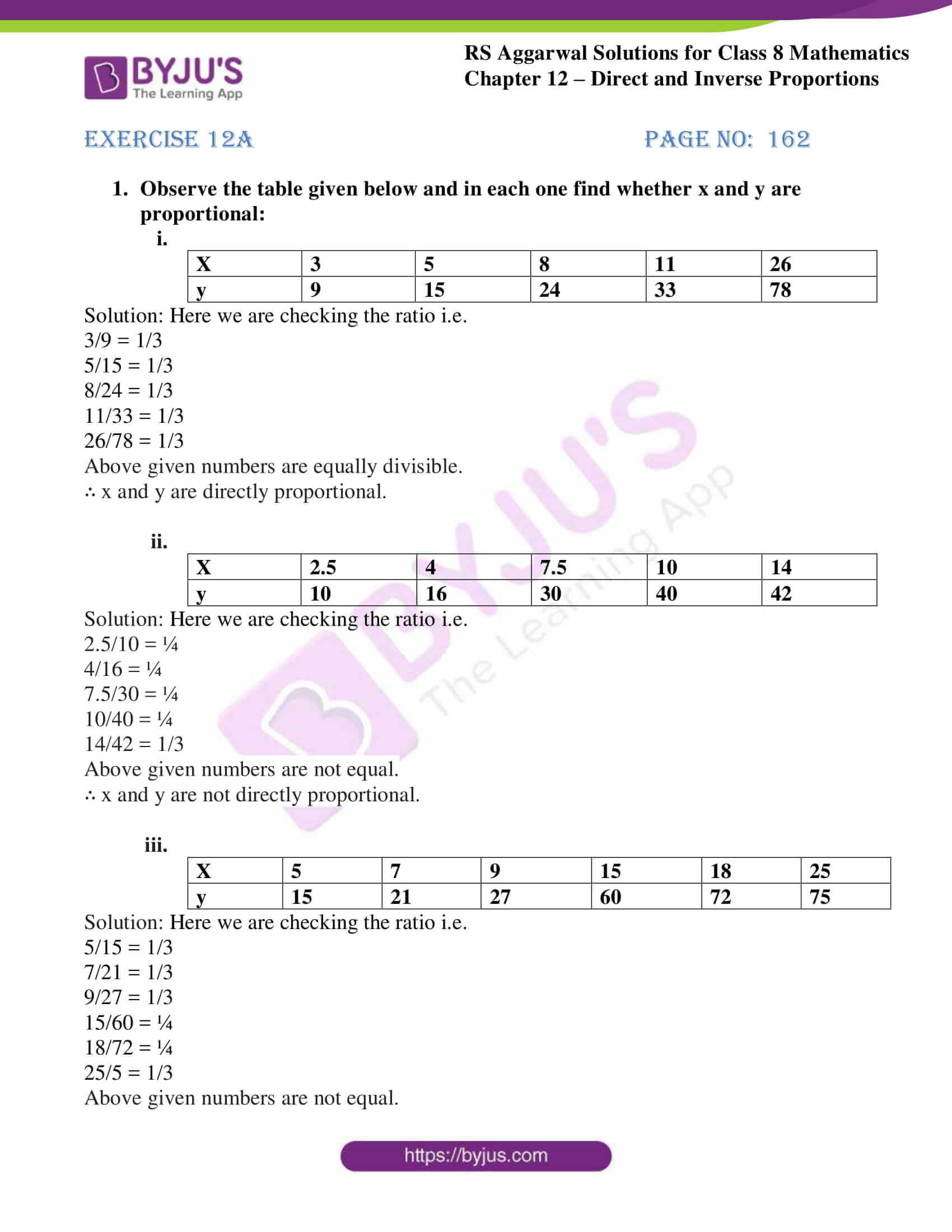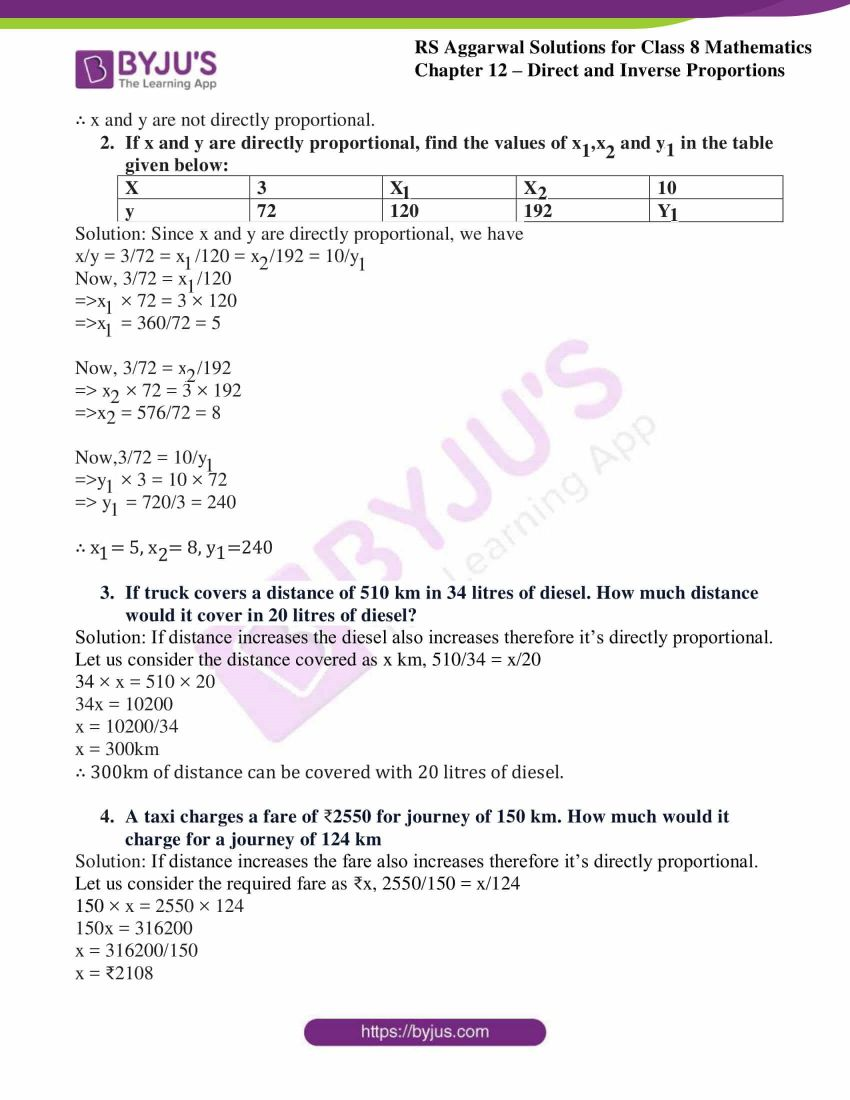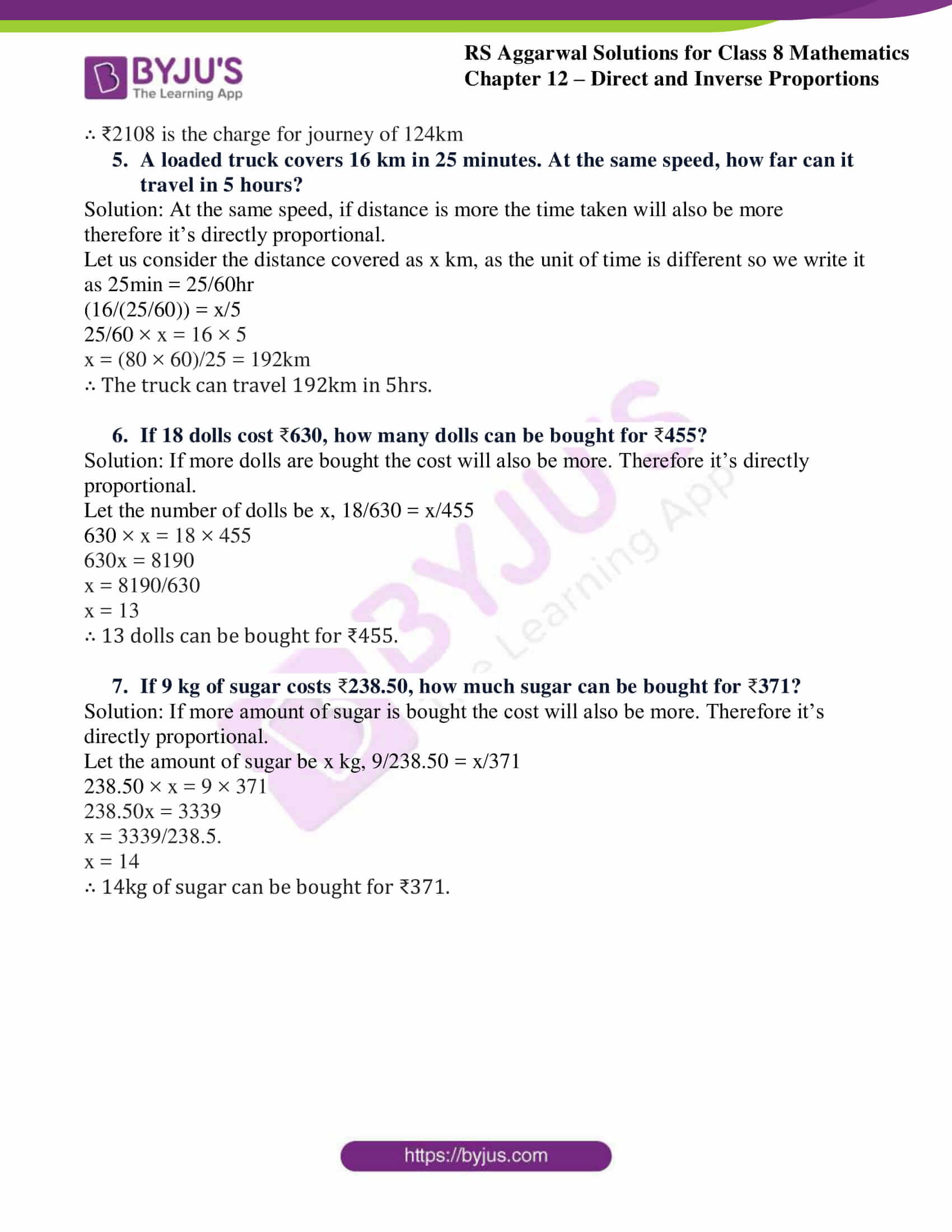# RS Aggarwal Solutions for Class 8 Maths Chapter 12 - Direct and Inverse Proportions Exercise 12A

Students can refer and download RS Aggarwal Solutions for Class 8 Maths Chapter 12- Exercise 12A, Direct and Inverse Proportions from the links provided below. Our experts have solved the RS Aggarwal Solutions to ensure that the students are thorough with their basic concepts by practicing the solutions.

In Exercise 12A of RS Aggarwal Class 8 Maths, we shall learn about Variation and Direct Proportion.

## Download PDF of RS Aggarwal Solutions for Class 8 Maths Chapter 12- Direct and Inverse Proportions Exercise 12A### Access Answers to RS Aggarwal Solutions for Class 8 Maths Chapter 12- Direct and Inverse Proportions Exercise 12A

1. Observe the table given below and in each one find whether x and y are proportional:
(i).

 X 3 5 8 11 26 y 9 15 24 33 78

Solution: Here we are checking the ratio i.e.

3/9 = 1/3

5/15 = 1/3

8/24 = 1/3

11/33 = 1/3

26/78 = 1/3

Above given numbers are equally divisible.

∴ x and y are directly proportional.

(ii).

 X 2.5 4 7.5 10 14 y 10 16 30 40 42

Solution: Here we are checking the ratio i.e.

2.5/10 = ¼

4/16 = ¼

7.5/30 = ¼

10/40 = ¼

14/42 = 1/3

Above given numbers are not equal.

∴ x and y are not directly proportional.

(iii).

 X 5 7 9 15 18 25 y 15 21 27 60 72 75

Solution: Here we are checking the ratio i.e.

5/15 = 1/3

7/21 = 1/3

9/27 = 1/3

15/60 = ¼

18/72 = ¼

25/5 = 1/3

Above given numbers are not equal.

∴ x and y are not directly proportional.

2. If x and y are directly proportional, find the values of x1,x2 and y1 in the table given below:

 x 3 x1 x2 10 y 72 120 192 y1

Solution: Since x and y are directly proportional, we have

x/y = 3/72 = x1/120 = x2/192 = 10/y1

Now, 3/72 = x1/120

⇨ x1 × 72 = 3 × 120

⇨ x1 = 360/72 = 5

Now, 3/72 = x2/192

⇨ x2 × 72 = 3 × 192

⇨ x2 = 576/72 = 8

Now,3/72 = 10/y1

⇨ y1 × 3 = 10 × 72

⇨ y1 = 720/3 = 240

∴ x1= 5, x2= 8, y1=240

3. If truck covers a distance of 510 km in 34 litres of diesel. How much distance would it cover in 20 litres of diesel?

Solution: If distance increases the diesel also increases therefore it’s directly proportional.

Let us consider the distance covered as x km, 510/34 = x/20

34 × x = 510 × 20

34x = 10200

x = 10200/34

x = 300km

∴ 300km of distance can be covered with 20 litres of diesel.

4. A taxi charges a fare of 2550 for journey of 150 km. How much would it charge for a journey of 124 km

Solution: If distance increases the fare also increases therefore it’s directly proportional.

Let us consider the required fare as ₹x, 2550/150 = x/124

150 × x = 2550 × 124

150x = 316200

x = 316200/150

x = ₹2108

∴ ₹2108 is the charge for journey of 124km

5. A loaded truck covers 16 km in 25 minutes. At the same speed, how far can it travel in 5 hours?

Solution: At the same speed, if distance is more the time taken will also be more therefore it’s directly proportional.

Let us consider the distance covered as x km, as the unit of time is different so we write it as 25min = 25/60hr

(16/(25/60)) = x/5

25/60 × x = 16 × 5

x = (80 × 60)/25 = 192km

∴ The truck can travel 192km in 5hrs.

6. If 18 dolls cost 630, how many dolls can be bought for 455?

Solution: If more dolls are bought the cost will also be more. Therefore it’s directly proportional.

Let the number of dolls be x, 18/630 = x/455

630 × x = 18 × 455

630x = 8190

x = 8190/630

x = 13

∴ 13 dolls can be bought for ₹455.

7. If 9 kg of sugar costs 238.50, how much sugar can be bought for 371?

Solution: If more amount of sugar is bought the cost will also be more. Therefore it’s directly proportional.

Let the amount of sugar be x kg, 9/238.50 = x/371

238.50 × x = 9 × 371

238.50x = 3339

x = 3339/238.5.

x = 14

∴ 14kg of sugar can be bought for ₹371.

### Access other Exercises of RS Aggarwal Solutions for Class 8 Maths Chapter 12- Direct and Inverse Proportions

Exercise 12B Solutions 15 Questions

Exercise 12C Solutions 10 Questions

## RS Aggarwal Solutions for Class 8 Maths Chapter 12- Direct and Inverse Proportions Exercise 12A

Exercise 12A of RS Aggarwal Class 8, Direct and Inverse Proportions. This exercise mainly deals with Variation- If two quantities depend on each other in such a way that the change in one results in a corresponding change in the other, then the two quantities are said to be in variation. Direct Proportion- Two quantities x and y are said to be in direct proportion if whenever the value of x increases (or decreases), then the value of y increases (or decreases) in such a way that the ratio x/y remains constant.

Students are suggested to practice the problems on a regular basis which will help them excel in their exams and increase their overall percentage. Practicing as many times as possible helps in building time management skills and also boosts the confidence level to achieve high marks.